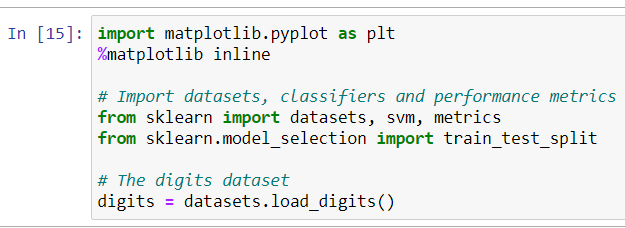# R

Original article was published by Kumar Vaibhav on Artificial Intelligence on Medium# Recognizing Handwritten Digits with scikit-learn

Recognizing handwritten text is a problem that can be traced back to the first automatic machines that needed to recognize individual characters in handwritten documents. Classifying handwritten text or numbers is important for many real-world scenarios. For example, a postal service can scan postal codes on envelopes to automate the grouping of envelopes which has to be sent to the same place. This article presents recognizing the handwritten digits (0 to 9) using the famous digits data set from Scikit-Learn, using a classifier called Logistic Regression.

Let us start by importing our libraries

Now we will visualize the training data

Creating a supporting classifier.

now we will predict the value of digit.

A confusion matrix is a table that is often used to evaluate the accuracy of a classification model. We can use Seaborn or Matplotlib to plot the confusion matrix. We will be using for Matplotlib our confusion matrix.

From this article, we can see how easy it is to import a dataset, build a model using Scikit-Learn, train the model, make predictions with it, and finding the accuracy of our prediction(which in our case is 97.11%). I hope this article helps you with your future endeavors!